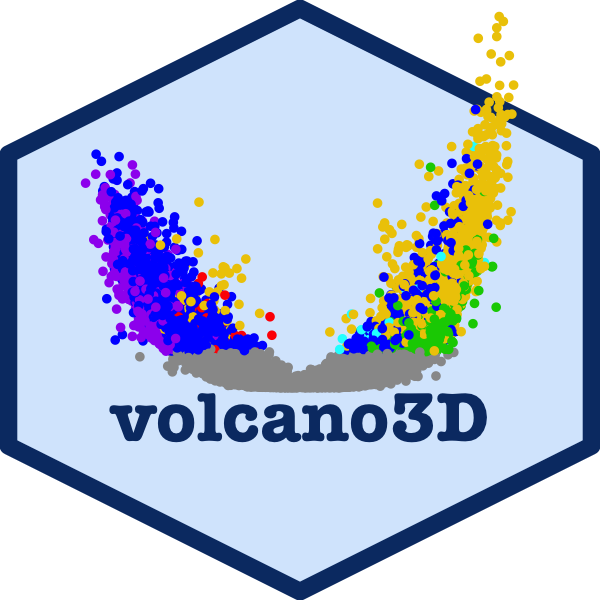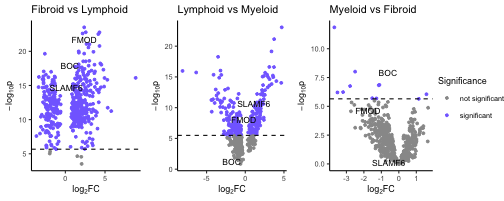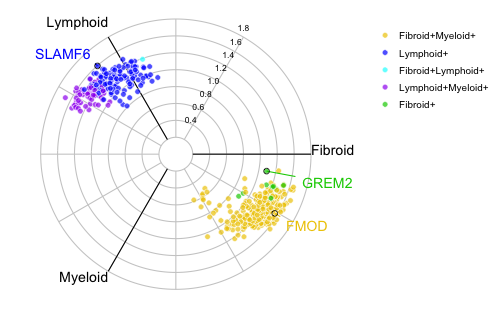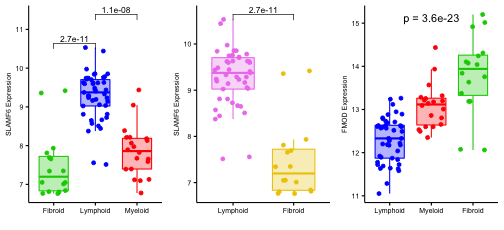# volcano3DThe volcano3D package enables exploration of probes differentially expressed between three groups. Its main purpose is for the visualisation of differentially expressed genes in a three-dimensional volcano plot. These plots can be converted to interactive visualisations using plotly.

This vignette covers the basic features of the package using a small example data set. To explore more extensive examples and view the interactive radial and volcano plots, see the extended vignette which explores a case study from the PEAC rheumatoid arthritis trial (Pathobiology of Early Arthritis Cohort). The methodology has been published in Lewis, Myles J., et al. Molecular portraits of early rheumatoid arthritis identify clinical and treatment response phenotypes. Cell reports 28.9 (2019): 2455-2470. (DOI: 10.1016/j.celrep.2019.07.091) with an interactive, searchable web tool available at https://peac.hpc.qmul.ac.uk. This was creating as an R Shiny app and deployed to the web using a server.

There are also supplementary vignettes with further information on:

## Getting Started

### Install from CRAN

``````install.packages("volcano3D")
``````

### Install from Github

``````library(devtools)
install_github("KatrionaGoldmann/volcano3D")
``````

``````library(volcano3D)
``````

# Dictionary

Variables used in this vignette:

Variable Definition
contrast the variable by which samples can be split into three groups.
groups the three levels/categories of the contrast variable. These should not contain underscores.
comparison two groups between which a statistical test can be performed. There should be three comparisons total. For the examples outlined in this vignette we look at comparisons: 'lymphoid-myeloid', 'lymphoid-fibroid' and 'myeloid-fibroid'.
p p value
FC fold change
suffix the tail word in a column name. In this package it states the statistical parameter (e.g. logFC is the log FC variable).
prefix the leading word in a column name. In this package it states the statistical test (e.g. LRT is the likelihood ratio test).
polar A polar coordinates object, of S4 class, containing the expression data, sample data, pvalues and polar coordinates.

# Examples

This vignette uses a subset of the 500 most highly differentially expressed genes from the PEAC data to explore the package functions. This can be loaded using:

``````data("example_data")
``````

Which contains:

• syn_example_rld - the log transformed expression data

• syn_example_p - the pvalues data frame containing differential expression statistics about each gene

• syn_example_meta which contains information about each sample.

Samples in this cohort fall into three pathotype groups:

``````kable(table(syn_example_meta\$Pathotype), col.names = c("Pathotype", "Count"))
``````
Pathotype Count
Fibroid 16
Lymphoid 45
Myeloid 20

These will be used as the differential expression groups for the three-way analysis.

## Creating Polar Coordinates

First, the differential expression can be mapped to polar coordinates using the polar_coords function, which has inputs:

Variable Details
sampledata (required) This shows information for each sample in rows and must contain: * an ID column: Containing the sample IDs. This must be titled ‘ID’. * a contrast column: Indicates which of the three groups each sample belongs to.
contrast (required) The column name in sampledata which contains the three-level factor to be used for contrast
pvalues (required) the pvalues data frame which contains the statistical significance of probes between groups. This contains: * three pvalue columns: one for each comparison with column names of format paste(groups[i], groups[j], p_col_suffix, sep=‘_’). We recommend using ‘limma’ or ‘DESeq’ pipelines to calculate these pvalues for gene expression. * _optional_ fold change columns: one for each comparison with column names of format paste0(groups[i], groups[j], fc_col_suffix, sep=‘_’) * _optional_ adjusted pvalue columns: one for each comparison with column names of format paste0(groups[i], groups[j], padj_col_suffix, sep=‘_’) * an _optional_ multi-group pvalue column: from a multi-group test with column name of the form paste0(multi_group_prefix, ‘_’, p_col_suffix).This is typically generated using ANOVA or likelihood ratio tests between all three groups. * an _optional_ multi-group adjusted pvalue column: from a multi-group test (column names of form paste0(multiGroupPrefix, ‘_’, padjColSuffix)). For more information on how to create pvalues data frames see the [pvalue generator vignette](https://katrionagoldmann.github.io/volcano3D/articles/pvalues_generator.html).
expression (required) A data frame or matrix containing the expression data. This is used to calculate z-score and fold change, therefore it should be a normalised expression object such as log transformed or variance stabilised counts.
groups The groups to be compared (in order). If NULL this defaults to levels(sampledata[, ‘contrasts’]). These must not contain underscores.
p_col_suffix The suffix of column names with pvalues (default is ‘pvalue’). This must not contain underscores.
padj_col_suffix The suffix of column names with adjusted pvalues (default is ‘padj’). This must not contain underscores. If NULL the adjusted pvalue is calculated using p_col_suffix and pvalue_method.
padjust_method The method to calculate adjusted pvalues if not already provided. Must be one of c(‘holm’, ‘hochberg’, ‘hommel’, ‘bonferroni’, ‘BH’, ‘BY’, ‘fdr’, ‘none’). Default is ‘BH’.
fc_col_suffix The suffix of column names with log(fold change) values (default is ‘logFC’). This must not contain underscores.
multi_group_prefix The prefix for columns containing statistics for a multi-group test (this is typically a likelihood ratio test or ANOVA). Default is NULL. This must not contain underscores.
label_column A column name in pvalues which is to be used to label markers of interest at plotting stage. If NULL the rownames will be used.

This can be applied to the example data as below:

``````data("example_data")

syn_polar <- polar_coords(sampledata = syn_example_meta,
contrast = "Pathotype",
pvalues = syn_example_p,
expression = syn_example_rld,
p_col_suffix = "pvalue",
fc_col_suffix = "log2FoldChange",
multi_group_prefix = "LRT",
non_sig_name = "Not Significant",
significance_cutoff = 0.01,
label_column = NULL,
fc_cutoff = 0.1)
``````

This creates an polar class object with slots for: sampledata, contrast, pvalues, multi_group_test, expression, polar and non_sig_name. The pvalues slot should now have at least two statistics for each comparison - pvalue and adjusted pvalue - and an optional logarithmic fold change statistic:

``````head(syn_polar@pvalues)
``````
Fibroid_Lymphoid _pvalue Fibroid_Lymphoid _logFC Fibroid_Lymphoid _padj Lymphoid_Myeloid _pvalue Lymphoid_Myeloid _logFC Lymphoid_Myeloid _padj Myeloid_Fibroid _pvalue Myeloid_Fibroid _logFC Myeloid_Fibroid _padj LRT _pvalue LRT _padj label
FMOD 0 2.377676 0 3.0e-07 -1.096532 0.0052783 0.0000021 -1.2811444 0.0332583 0 0 FMOD
KCNIP3 0 2.575418 0 0.0e+00 -1.538422 0.0000013 0.0004665 -1.0369961 1.0000000 0 0 KCNIP3
TRIM29 0 4.301344 0 0.0e+00 -3.515507 0.0000000 0.1083911 -0.7858377 1.0000000 0 0 TRIM29
CILP 0 4.159372 0 0.0e+00 -2.388539 0.0000083 0.0002431 -1.7708331 1.0000000 0 0 CILP
CAB39L 0 2.176451 0 4.0e-07 -1.022201 0.0055897 0.0000046 -1.1542502 0.0740328 0 0 CAB39L
PAMR1 0 2.666598 0 7.3e-06 -1.144613 0.1126032 0.0000020 -1.5219853 0.0321042 0 0 PAMR1

The `sig` column in `syn_polar@polar` allows us to determine relative differences in expression between groups (in this case pathotypes). The '+' indicates which pathotypes are significantly 'up' compared to others. For example:

• genes labelled 'Lymphoid+' are significantly up in Lymphoid vs Myeloid and Lymphoid vs Fibroid.

• genes up in two pathotypes such as 'Lymphoid+Myeloid+' are up in both Lymphoid and Myeloid, therefore Lymphoid vs Fibroid and Myeloid vs Fibroid are statistically significant.

• genes which show no significant difference between pathotypes are classed according to `non_sig_name`

This gives us:

``````setNames(data.frame(table(syn_polar@polar\$sig)), c("Significance", "Frequency"))
``````
Significance Frequency
Fibroid+ 9
Fibroid+Lymphoid+ 1
Fibroid+Myeloid+ 310
Lymphoid+ 124
Lymphoid+Myeloid+ 56

## Volcano Plots

If a fold change column was previously provided, the comparisons between pathotypes can be investigated using the volcano_trio function. This creates ggplot outputs:

``````syn_plots <- volcano_trio(polar = syn_polar,
sig_names = c("not significant","significant",
"not significant","significant"),
colours = rep(c("grey60",  "slateblue1"), 2),
text_size = 9,
marker_size=1,
shared_legend_size = 0.9,
label_rows = c("SLAMF6", "BOC", "FMOD"),
fc_line = FALSE,
share_axes = FALSE)

syn_plots\$All
``````The differential expression can now be visualised on an interactive radial plot using radial_plotly.

``````radial_plotly(polar = syn_polar, label_rows = c("SLAMF6", "GREM2", "FMOD"),
grid_colour="pink", axis_colour="blue")
``````Unfortunately CRAN does not support interactive plotly in the vignette, but these can be viewed on the extended vignette. When interactive, it is possible to identify genes for future interrogation by hovering over certain markers.

Similarly a static ggplot image can be created using radial_ggplot:

``````radial_ggplot(polar = syn_polar,
label_rows = c("SLAMF6", "FMOD", "GREM2"),
marker_size = 2.3,
legend_size = 10) +
theme(legend.position = "right")
``````## Boxplots

Any one specific probe can be interrogated using a boxplot to investigate differences between groups:

``````plot1 <- boxplot_trio(syn_polar,
value = "SLAMF6",
text_size = 7,
my_comparisons=list(c("Lymphoid", "Myeloid"),
c("Lymphoid", "Fibroid")))

plot2 <- boxplot_trio(syn_polar,
value = "SLAMF6",
box_colours = c("violet", "gold2"),
levels_order = c("Lymphoid", "Fibroid"),
text_size = 7,
)

plot3 <- boxplot_trio(syn_polar,
value = "FMOD",
text_size = 7,
stat_size=2.5,
levels_order = c("Lymphoid", "Myeloid", "Fibroid"),
box_colours = c("blue", "red", "green3"))

ggarrange(plot1, plot2, plot3, ncol=3)
``````## Three Dimensional Volcano Plots

Lastly, the 3D volcano plot can be used to project differential gene expression onto cylindrical coordinates. Again, CRAN does not support WebGL for the interactive 3D plot, however this can be viewed in the extended vignette.

``````p <- volcano3D(syn_polar,
label_rows = c("SLAMF6", "GREM2", "FMOD"),
label_size = 10,
xy_aspectratio = 1,
z_aspectratio = 0.9,
plot_height = 700)
p
``````## Saving Plotly Plots

### Static Images

There are a few ways to save plotly plots as static images. Firstly plotly offers a download button ( ) in the figure mode bar (appears top right). By default this saves images as png, however it is possible to convert to svg, jpeg or webp using:

``````p %>% plotly::config(toImageButtonOptions = list(format = "svg"))
``````

Alternatively, if orca command-line utility is installed, this can also be used to save plotly images. To install follow the instructions here.

``````orca(p, "./volcano_3d_synovium.svg", format = "svg")
``````

### Interactive HTML

The full plotly objects can be saved to HTML by converting them to widgets and saving with the htmlwidgets package:

``````htmlwidgets::saveWidget(as_widget(p), "volcano3D.html")
``````

# Citation

volcano3D was developed by the bioinformatics team at the Experimental Medicine & Rheumatology department and Centre for Translational Bioinformatics at Queen Mary University London.

If you use this package please cite as:

``````citation("volcano3D")
``````
``````##
## To cite package 'volcano3D' in publications use:
##
##   Katriona Goldmann and Myles Lewis (2020). volcano3D: Interactive
##   Plotting of Three-Way Differential Expression Analysis.
##   https://katrionagoldmann.github.io/volcano3D/index.html,
##   https://github.com/KatrionaGoldmann/volcano3D.
##
## A BibTeX entry for LaTeX users is
##
##   @Manual{,
##     title = {volcano3D: Interactive Plotting of Three-Way Differential Expression
## Analysis},
##     author = {Katriona Goldmann and Myles Lewis},
##     year = {2020},
##     note = {https://katrionagoldmann.github.io/volcano3D/index.html, https://github.com/KatrionaGoldmann/volcano3D},
##   }
``````

or using:

Lewis, Myles J., et al. Molecular portraits of early rheumatoid arthritis identify clinical and treatment response phenotypes. Cell reports 28.9 (2019): 2455-2470.